There are rules when doing math in elementary school. You cannot do the calculations any way you like. There are rules for calculations, and you have to follow the order of the rules.

In elementary arithmetic, what are the rules when doing calculations? One of the most important rules is that multiplication and division should be done first. Another rule is that the numbers in parentheses must be calculated first.

Why are these rules in place? And how should we do the calculations? In this section, we will explain the rules of the four arithmetic operations.

## There Is an Order to Math Calculations

There is an order to calculations. Therefore, we need to learn the rules of calculation. Addition, subtraction, multiplication, and division are called the four arithmetic operations. If you do not understand the rules of the four arithmetic operations, you will not be able to calculate correctly.

For example, the answers to the following questions will be different.

• $2+3×6-1=19$
• $(2+3)×6-1=29$
• $(2+3)×(6-1)=25$

The numbers are the same. Nevertheless, the answer changes depending on the parentheses. The reason why you should understand the order of operations is that you can’t get the answer.

Normally, in arithmetic, we calculate in order from left to right. However, in some cases, we must not calculate in order from left to right. The following are the rules for the order of calculation in the four arithmetic operations that should be learned in elementary math.

• Give priority to multiplication and division.
• Calculate inside the parentheses first.

Let’s check the details of each.

### Give Priority to Multiplication and Division

In arithmetic operations, addition, subtraction, multiplication, and division are mixed. Of these, multiplication and division are given priority over addition and subtraction. This means that if there is a multiplication or division symbol, it must be calculated first.

For example, what is the answer to the following equation?

• $3+2×4$

In the order of operation, multiplication takes precedence over addition. Therefore, instead of calculating from left to right, multiplication has to be done first. So calculate $2×4=8$ first.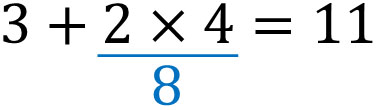After that, add 3 and 8. This gives us 11 as the answer. In this way, multiplication and division have priority when doing calculations.

Also, what about the following calculation?

• $20÷4-2×2$

In the same way, give priority to multiplication and division over subtraction in this calculation. The result is as follows.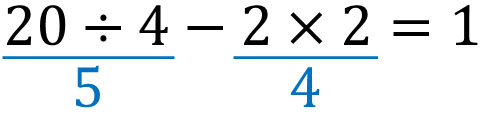If we calculate multiplication and division first, $20÷4=5$ and $2×2=4$. Therefore, the answer is 1 by subtracting 4 from 5.

### Calculating Inside Parentheses First

Although multiplication and division take precedence in calculations, there is an exception. The exception to this is parentheses. If there are parentheses, even if there is a multiplication or division symbol, there is a rule that the inside of the parentheses must be calculated first.

In the calculation, the inside of the parentheses is considered as a single number. Therefore, the rule is that the number inside the parentheses must be calculated first. For example, it goes like this.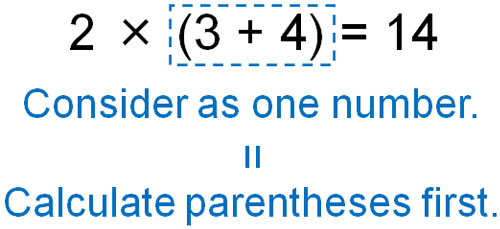Normally, multiplication and division take precedence. However, in this example, since there are parentheses, addition is done first.

### Calculating with Multiplication and Division in Parentheses

Now, let’s try a more complicated calculation. For example, how do we calculate the following?

• $20-(1+6÷2)×(1+2)$

First, calculate the inside of the parentheses. However, there is a division inside the parentheses. So we must calculate the division inside the parentheses first.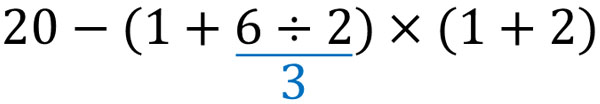There is a common rule that multiplication and division take precedence over addition and subtraction. Therefore, calculate $6÷2=3$ first.

After that, do the addition in parentheses. The result is as follows.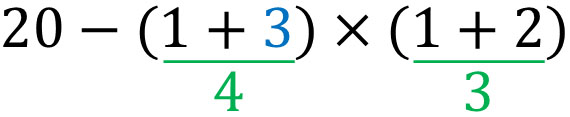By calculating inside the parentheses, we get the answers $1+3=4$ and $1+2=3$. In the next calculation, multiplication takes precedence. Therefore, let’s do multiplication instead of subtraction.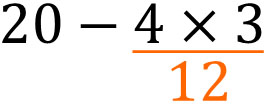In this way, calculate $4×3=12$ first. Then, we can get the answer by subtracting $20-12=8$.

## Why Are There Rules for Calculations in the Four Arithmetic Operations?

Why are there rules in the four arithmetic operations? Also, why do we use parentheses? This is because, without rules, calculations cannot be done correctly.

For example, let’s consider the following situation.

• There is a cake that costs \$3 and a donut that costs \$2. If you buy four sets, how much will they cost?There is a cake and a donut on sale, and when you add the two together, you get $3+2=5$. Since you are buying four sets of these items that cost \$5 each, we need to multiply them. Therefore, we need to consider$3+2$as a single number. The equation is as follows. •$(3+2)×4\$

If there are no parentheses, the multiplication will be calculated first, and the correct answer cannot be obtained. If you add parentheses, you can calculate the addition first instead of the multiplication. If you understand the order of operations, you will be able to create the correct expression and give the correct answer.

The more detailed rules of the four arithmetic operations are learned in advanced mathematics such as junior high school. Therefore, when you learn more about math, you can study the reasons for the order of operations in more detail.

### Numbering Reduces Miscalculations

Although the four arithmetic operations have the order of operations, if you are not familiar with them, you may calculate in order from left to right. Therefore, if you are not used to it, you should number the calculations first. By numbering the order of calculations, you will be able to prevent miscalculation.

Let’s number them as follows.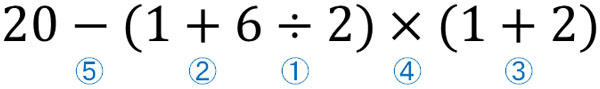If you can number them correctly, you will be able to calculate them in the correct order.

Until you get used to it, it is important not to make any calculation mistakes. Understanding the order of operations is very important in arithmetic, so be sure to remember it.

## Learning the Rules of Calculation in Elementary Arithmetic

Arithmetic is used frequently in our daily lives. For example, when we buy something, we always calculate the number.

If you don’t understand the order of operations, you won’t be able to calculate correctly. Why do we have rules for the four arithmetic operations? It is because, without rules, it is impossible to get the correct answer.

Understand that there are reasons for the order of operations when doing math calculations. If you are not familiar with it, you can write down the numbers first. In any case, try to remember the rules of calculation.